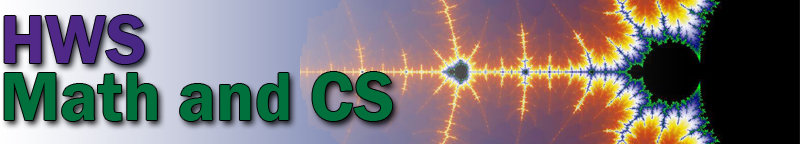Schedule of Courses for Fall 2021 Period 1 MWF 8:40–9:40 Math 130: Calculus I, Jocelyn Bell       (Lab Tuesday, 8:30–10:00) CPSC 124: Introductory Programming, Hanqing Hu       (Lab Thursday, 11:50–1:20) Period 2 MWF 9:50–10:50 Math 130: Calculus I, Jocelyn Bell       (Lab Tuesday, 10:10–11:40) Math 131: Calculus II, Alden Gassert       (Lab Tuesday, 11:50–1:20) CPSC 124: Introductory Programming, David Eck       (Lab Tuesday, 10:10–11:40) Period 3 MWF 11:00–12:00 Math 130: Calculus I, Erika King       (Lab Thursday, 10:10–11:40) Math 232: Multivariable Calculus, Jennifer Biermann CPSC 371: Advanced Topics in Computer Science, Hanqing Hu Period 4 MWF 12:10–1:10 Math 130: Calculus I, Erika King       (Lab Thursday, 11:50–1:20) Math 214: Applied Linear Algebra, Alden Gassert CPSC 329: Software Development, Chris Fietkiewicz       (Lab Tuesday, 8:30–10:00) Period 5 MWF 1:20–2:20 Math 100: Elementary Functions, Jonathan Forde       (Lab Tuesday, 11:50–1:20) CPSC 424: Computer Graphics, David Eck       (Lab Thursday, 10:10–11:40) Period 5A MW 1:20–2:50 Math 204: Linear Algebra, Jocelyn Bell Period 6 MW 3:00–4:30 Math 135: First Steps into Advanced Math, Jennifer Biermann Period 8 TuTh 10:10–11:40 Math 353: Mathematical Models, Yan Hao Period 10 TuTh 1:30–3:00 Math 110: Discovering in Mathematics, Yan Hao CPSC 220: Computer Architecture, Chris Fietkiewicz       (Lab Friday, 9:50–10:50) Period 11 TuTh 3:10–4:40 Math 331: Foundations of Analysis, Jonathan Forde

 Tentative Schedule of Courses for Spring 2022 Period 1 MWF 8:40–9:40 Math 131: Calculus II, Jennifer Biermann       (Lab Thursday, 11:50–1:20) Period 1A MW 9:20–10:50 Math 204: Linear Algebra, Yan Hao Period 2 MWF 9:50–10:50 Math 130: Calculus I, Alden Gassert       (Lab Tuesday, 11:50–1:20) Math 131: Calculus II, Erika King       (Lab Thursday, 1:30–3:00) CPSC 225: Intermediate Programming, Stina Bridgeman       (Lab Tuesday, 11:50–1:20) Period 3 MWF 11:00–12:00 Math 130: Calculus I, Alden Gassert       (Lab Tuesday, 10:10–11:40) Math 375: Abstract Algebra, Jennifer Biermann CPSC 229: Foundations of Computation, Hanqing Hu Period 4 MWF 12:10–1:10 Math 130: Calculus I, Jonathan Forde       (Lab Tuesday, 1:30–3:00) CPSC 327: Data Structures and Algorithms, Stina Bridgeman Period 5 MWF 1:20–2:20 Math 232: Multivariable Calculus, Jonathan Forde CPSC 124: Introductory Programming, Hanqing Hu       (Lab Tuesday, 1:30–3:00) CPSC 331: Operating Systems, Chris Fietkiewicz Period 5A MW 1:20–2:50 Math 237: Differential Equations, Yan Hao Period 8 TuTh 10:10–11:40 Math 135: First Steps into Advanced Math, Jocelyn Bell CPSC 433: Compilers, Chris Fietkiewicz       (Lab Friday, 9:20–10:50) Period 9 TuTh 11:50–1:20 Math 313: Graph Theory, Erika King Period 10 TuTh 1:30–3:00 Math 471: Mathematics Capstone, Jocelyn Bell